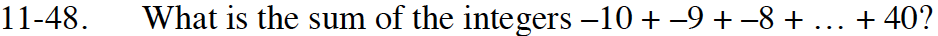### Home > INT3 > Chapter 11 > Lesson 11.1.4 > Problem11-48

11-48.

What is the sum of the integers –10 + –9 + –8 + … + 40? Homework Help ✎Remember the summation formula:

$S=\frac{n(t(1)+t(n))}{2}$

765